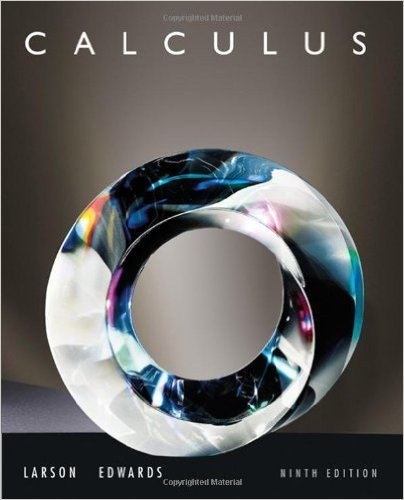×
×

# Solutions for Chapter 8.5: Partial Fractions## Full solutions for Calculus | 9th Edition

ISBN: 9780547167022Solutions for Chapter 8.5: Partial Fractions

Solutions for Chapter 8.5
4 5 0 281 Reviews
14
2
##### ISBN: 9780547167022

Chapter 8.5: Partial Fractions includes 70 full step-by-step solutions. Calculus was written by and is associated to the ISBN: 9780547167022. This expansive textbook survival guide covers the following chapters and their solutions. Since 70 problems in chapter 8.5: Partial Fractions have been answered, more than 64179 students have viewed full step-by-step solutions from this chapter. This textbook survival guide was created for the textbook: Calculus , edition: 9.

Key Calculus Terms and definitions covered in this textbook
• Bounded above

A function is bounded above if there is a number B such that ƒ(x) ? B for all x in the domain of ƒ.

• DMS measure

The measure of an angle in degrees, minutes, and seconds

• Domain of validity of an identity

The set of values of the variable for which both sides of the identity are defined

• Higher-degree polynomial function

A polynomial function whose degree is ? 3

• Independent events

Events A and B such that P(A and B) = P(A)P(B)

• Initial value of a function

ƒ 0.

• Integers

The numbers . . ., -3, -2, -1, 0,1,2,...2

• Integrable over [a, b] Lba

ƒ1x2 dx exists.

• Inverse cosecant function

The function y = csc-1 x

• Logarithmic re-expression of data

Transformation of a data set involving the natural logarithm: exponential regression, natural logarithmic regression, power regression

• LRAM

A Riemann sum approximation of the area under a curve ƒ(x) from x = a to x = b using x1 as the left-hand endpoint of each subinterval

A measure that tells how widely distributed data are.

• Outliers

Data items more than 1.5 times the IQR below the first quartile or above the third quartile.

• Perihelion

The closest point to the Sun in a planet’s orbit.

• Pythagorean

Theorem In a right triangle with sides a and b and hypotenuse c, c2 = a2 + b2

The measure of an angle in radians, or, for a central angle, the ratio of the length of the intercepted arc tothe radius of the circle.

• Reciprocal of a real number

See Multiplicative inverse of a real number.

• Stem

The initial digit or digits of a number in a stemplot.

• Symmetric difference quotient of ƒ at a

ƒ(x + h) - ƒ(x - h) 2h

• Vertex of a parabola

The point of intersection of a parabola and its line of symmetry.

×